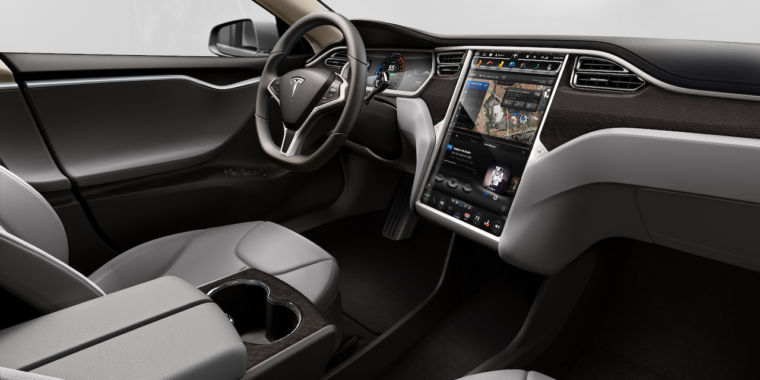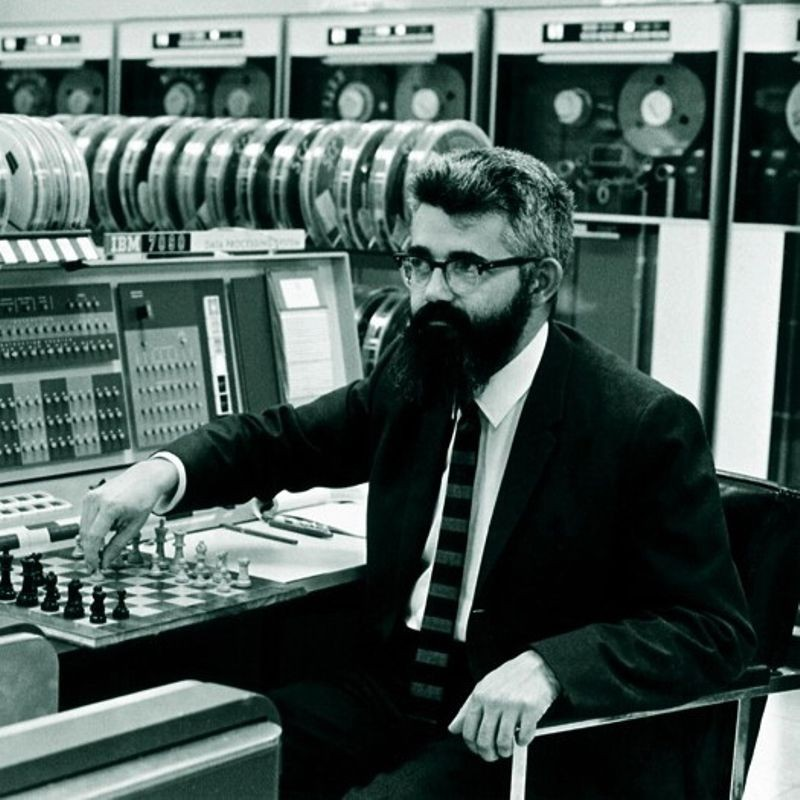# Learning¶

In this notebook we'll discuss what it means for a computer to learn.

## Function¶The term function is often not clearly defined and has many different uses.

In programming, we usually write functions where we know exactly what the return value should be for given inputs.

We also know the precise, basic steps the function should take in sequence to determine the return value.

For instance, consider the following function to calculate the greatest common divisor of two numbers.

In :
def gcd(a, b):
"""Calculate the Greatest Common Divisor of the integers a and b."""
# b evaluates as true unless it is zero.
while b:
# Set the new value of a to the old value of b, and the new value of
# b to the old value of a modulo the old value of a.
a, b = b, (a % b)
# Once b is zero, a is the GCD.
return a


The function, while not trivial, is straight-forward and precise.

It's clear that the inputs should be two numbers (natural numbers, in fact), and the return value will also be a (natural) number.

There is an infinity of natural numbers, so we can't write a list of all the inputs and their respective outputs.

However, in practice a computer can only store a limited amount of natural numbers of limited size.

In any case, it's clear we know a lot about the task of calculating the greatest common divisor.

## Driving a car¶Consider instead something quite complex: driving a car.

Can we write a function to do this task?

We'll suppose the function will be called on an infinite loop, say every 1000th of a second.

At each call the function should return the action to take to safely drive the car.

## Outputs¶The outputs of the function are straight-forward.

The user interface for a car mainly comprises of a steering wheel, an accelerator, and a brake.

We'll assume the car is an automatic and we won't worry about the handbrake or turning signals or lights.

We can measure the turning of the steering wheel in degrees, with zero degrees meaning the steering wheel is straight, -90 meaning the wheel has a quarter turn counter-clockwise and 90 meaning it has a quarter turn clockwise, etc.

We can measure each of the accelerator and brake in terms of how hard they are pressed on a linear scale from 0 to 100. If we are not pressing the pedal we'll say that's 0, and at a full press it's 100. 50 means we half press it.

So, the output of our function will be a triple, e.g. (-180, 0, 20) meaning turn the wheel to -180 degrees, don't press the accelerator at all, and press the break to 20%.

## Inputs¶

The inputs to the function are also somewhat straight-forward.

First, the current positions of the steering wheel, break and accelerator are relevant inputs. We don't want to go from 100 on the accelerator to 100 on the break in 1 millisecond.

In fact, we probably need to use the array of positions from the past minute or so, as inputs to give a smooth driving experience. Either way, while it's a lot of data, it's not very complex.The main input you would imagine we need is the view from the driver's seat.

This can be provided by a digital camera (or an array of cameras).

The camera will give us a big array of pixels (millions of them). Each pixel will have a red value (say, between 0 and 255), a blue value, and a green value.

These are just small integers, albeit a lot of them.

We can go one step further in that we can use lots of sensors placed on the outside of the car to gauge how close the car is to outside objects, and again we'll got lots of numbers.

## Difficulties¶I believed in realism, as summarized by John McCarthy’s comment to the effect that if we worked really hard, we’d have an intelligent system in from four to four hundred years. - Marvin Minsky

Now we have two difficult (possibly impossible) problems.

First, when we say that we want the function to correctly drive the car, how do define correct. Different humans would drive the car differently. Any sensible answers will define a list of criteria, such as not crashing into walls or people, but won't be exact.

Second, should we be able to give a concrete description of correctness, how do we convert the inputs into those outputs?

In the machine learning community there seems to be an acceptance that there is not going to be a deterministically-based answer to these questions any time soon, and perhaps never.

## Supervised learning¶A computer program learns from experience E, with respect to some task T, and some performance measure P, if its performance on T as measured by P improves with experience E. - Tom Mitchell

We can sidestep these two difficulties using supervised learning.

We start with a set of inputs for which we know the outputs we want.

We then train a model with these input-output pairs.

We have to pick a model but we can pick a generic one that can be moulded to mimic a whole load of other functions depending on how we train it.

We train the model by giving it the known input-output pairs and programmatically tweaking the model parameters to give (at least approximately) the given output when provided with the given input.

Progress and Future of Artificial Intelligence AI 2017 CMU Machine Learning Chair Tom Mitchell

https://www.youtube.com/watch?v=0Xj0n-HYtAM

A talk by Tom Mitchel on AI.